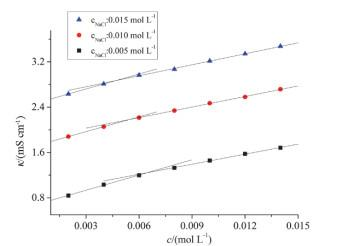## Study on the Physical and Chemical Properties of Surfactant Micellization

Li Yali,, Fan Jianxun, Wang Bingjie, Xiang Ling

 基金资助: 陕西省自然科学基础研究项目.  2021JQ-825渭南师范学院大学生创新创业训练计划项目.  19xk065Abstract

In this paper, the physical and chemical properties of micelle formation process of surfactants were studied by studying the influence of temperature and salt concentration on the critical micelle concentration (CMC) of sodium dodecyl sulfate (SDS) using conductivity method. The thermodynamic parameters of micelle formation were obtained according to the pseudo-phase separation model, and the conductance activation energy of the system dependence of temperature and SDS concentration was discussed. The results showed that CMC of SDS was increased with the increasing of temperature, and decreased with the increasing of sodium chloride concentration, suggesting a spontaneous, exothermic and entropy-increasing process in the micelle formation of SDS in aqueous solution; in kinetics, the relationship between the conductivity of the SDS solution and the temperature conforms to the Arrhenius formula, the activation energy information can reveal the mechanism of ionic surfactants forming micelles.

Keywords： Surfactant ; Critical micelle concentration ; Quasi-phase separation model ; Thermodynamics ; Activation energy

Li Yali. Study on the Physical and Chemical Properties of Surfactant Micellization. University Chemistry[J], 2022, 37(1): 2103039-0 doi:10.3866/PKU.DXHX202103039

### 图1### 1.2 胶束形成时热力学函数ΔHmӨ、ΔGmӨ和ΔSmӨ计算

$\mu_{\mathrm{s}}=\mu_{\mathrm{s}}^{\ominus}+R T \ln \gamma_{\mathrm{s}} \cdot \mathrm{CMC}$

$\mu_{\mathrm{s}}^{\mathrm{c}}=\mu_{\mathrm{m}}^{\ominus}=\mu_{\mathrm{S}}^{\ominus}+R T \ln \gamma_{\mathrm{s}} \cdot \mathrm{CMC}$

\begin{aligned}&\Delta G_{\mathrm{m}}^{\ominus}=\mu_{\mathrm{m}}^{\ominus}-\mu_{\mathrm{S}}^{\ominus}=R T \ln \mathrm{CMC} \\&\Delta G_{\mathrm{m}}^{\ominus}=R T \ln \mathrm{CMC}\end{aligned}

$\Delta G_{\mathrm{m}}^{\ominus}=R T \ln x_{\mathrm{S}}+(1-\alpha) R T \ln x_{\mathrm{X}}$

$\Delta G_{\mathrm{m}}^{\ominus}=(2-\alpha) R T \ln \mathrm{CMC}$

$\Delta G_{\mathrm{m}}^{\ominus}=2 R T \ln \mathrm{CMC}$

$\Delta H_{\mathrm{m}}^{\ominus}=-T^{2} \frac{\partial\left(\Delta H_{\mathrm{m}}^{\ominus} / T\right)}{\partial T}=-2 R T^{2} \frac{\partial \ln \mathrm{CMC}}{\partial T}$

$\Delta S_{\mathrm{m}}^{\ominus}=-2 R\left(\ln \mathrm{CMC}+T \frac{\partial \ln \mathrm{CMC}}{\partial T}\right)$

### 1.3 胶束形成时电导活化能

${\mathit{\Lambda }_{\rm{m}}} = {K_{\rm{V}}}/\eta$

$\eta=A_{\mathrm{V}} \exp \left(E_{\mathrm{V}} / R T\right)$

$\ln \mathit{\Lambda }_{\mathrm{m}}=\ln \left(K_{\mathrm{V}} / A_{\mathrm{V}}\right)-E_{\mathrm{V}} / R T$

$\ln {\mathit{\Lambda }_{\rm{m}}} = - {E_{\rm{V}}}/RT + {C^\prime }$

$\ln \kappa=-E_{\mathrm{V}} / R T+C$

### 2.1 仪器与试剂

HK-1D恒温水槽(南京大学应用物理研究所); DJS-1C电导率仪(上海雷磁创益仪器仪表有限公司); 101-2电热鼓风干燥箱(上海实验仪器总厂).

### 2.2 实验方法

(1) SDS溶液配制. SDS在80 ℃干燥3 h, 待冷却后称量, 用超纯水准确配制0.020 mol·L−1 SDS水溶液, 再稀释至0.002、0.004、0.006、0.008、0.010、0.012、0.014、0.016、0.018、0.020 mol·L−1备用.

(2) CMC测量. 打开电导率仪开关, 预热10 min. 在测定样品前, 用超纯水清洗并擦干电导率仪电极. 在25、40、60 ℃恒温条件下, 依次按浓度由低至高测量SDS溶液电导率, 分别测三次取平均值, 由电导率κ计算摩尔电导率Λm, 以κ-cΛm-c作图, 由其转折点处浓度确定CMC.

### 图2### 图3### 图4#### 3.2.1 胶束化Gibbs自由能ΔGmӨ

 T/℃ ΔGmӨ/(kJ∙mol−1) ΔHmӨ/(kJ∙mol−1) TΔSmӨ/kJ ΔSmӨ/(J∙K−1) 25 −23.386 −5.944 17.442 58.501 40 −28.802 −4.467 24.335 77.71 60 −25.606 −4.558 21.048 63.287

#### 3.2.2 胶束化焓变ΔHmӨ

$\ln \mathrm{CMC}=-6.19548+0.006970 T-6.7540 \times 10^{-6} T^{2}$

### 图5$\frac{\partial \ln \mathrm{CMC}}{\partial T}=0.006970-1.3508 \times 10^{-6} T$

#### 3.2.5 电导活化能

 c/(mol∙dm−3) lnκ-1/T线性方程 相关系数R2 电导活化能EV/(kJ∙mol−1) 0.0020 lnκ = −1950.366/T + 4.923 0.99999 16.215 0.0040 lnκ = −1968.240/T + 5.592 0.99996 16.364 0.0060 lnκ = −1937.278/T + 5.805 0.99911 16.106 0.0080 lnκ = −1957.256/T + 6.059 0.99871 16.273 0.0100 lnκ = −1948.512/T + 6.183 0.99951 16.120 0.0120 lnκ = −1948.512/T + 6.393 0.99977 16.450 0.0140 lnκ = −1974.971/T + 6.460 0.99972 16.420 0.0160 lnκ = −1966.106/T + 6.580 0.99949 16.346

 联系与区别 电导活化能EV 表观活化能Ea 理论依据 lnκ = −EV/RT + C lnk = −Ea/RT + lnA 求算方法 lnκ-1/T作图 lnk-1/T作图 直线斜率= −EV/R 直线斜率= −Ea/R 单位 J∙mol−1 J∙mol−1 物理意义 离子克服周围水分子和共存离子相互作用发生迁移所需的最低能量 由非活化分子变成活化分子所需要的能量 使用场合 离子迁移 化学反应

κ为电导率, k为速率常数

### 图6### 图7## 参考文献 原文顺序 文献年度倒序 文中引用次数倒序 被引期刊影响因子

Kallay N. ; Tomisic V. ; Hrust V. ; Pieri R. ; Chittofrati A. Colloid and Surface A 2003, 222, 95.

/

 〈〉